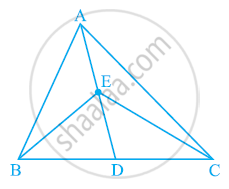# In the given figure, E is any point on median AD of a ΔABC. Show that ar (ABE) = ar (ACE) - Mathematics

In the given figure, E is any point on median AD of a ΔABC. Show that ar (ABE) = ar (ACE)#### Solution

AD is the median of ΔABC. Therefore, it will divide ΔABC into two triangles of equal areas.

∴ Area (ΔABD) = Area (ΔACD) ... (1)

ED is the median of ΔEBC.

∴ Area (ΔEBD) = Area (ΔECD) ... (2)

On subtracting equation (2) from equation (1), we obtain

Area (ΔABD) − Area (EBD) = Area (ΔACD) − Area (ΔECD)

Area (ΔABE) = Area (ΔACE)

Concept: Corollary: Triangles on the same base and between the same parallels are equal in area.
Is there an error in this question or solution?

#### APPEARS IN

NCERT Class 9 Maths
Chapter 9 Areas of Parallelograms and Triangles
Exercise 9.3 | Q 1 | Page 162